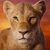# This Mental Math Test Is Almost Impossible to Pass, Let's See How You Do

## If you think you’re good at math, think again because this test is known to be almost impossible to pass!Created by Lea Abelson
On Apr 23, 2023

We know and we admit, algebra is hard but this test takes it to another level. Not many people can pass this quiz, are you a math genius and confident you can do it? These short math questions may seem easy at first glance, but look harder and it takes some skill. Let’s see how well you do on this near-impossible math test!

>> Score 7/10 and Your IQ is 145 or Higher <<

1 / 10

56 + 82 - 45 * 4 =

2 / 10

49 / 5 - 7 =

3 / 10

67 + 44 - 8 =

4 / 10

66 * 77 =

5 / 10

55 + 78 =

6 / 10

34 - 44 + 78 =

7 / 10

62 + 88 * 2 =

8 / 10

43 - 12 + 8 =

9 / 10

2.5 + 7 * 9 =

10 / 10

55 * 77 =

10
Questions left

### What is your favorite number?

Calculating results
These are 10 of the World CRAZIEST Ice Cream Flavors
Created by Tal Garner
On Nov 18, 2021